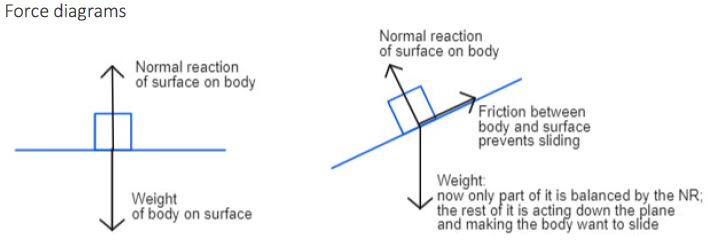# Equilibrium and resultant forces (A-level Maths Year 1)

This article, on equilibrium and resultant forces, follows on from my recent post on Forces and Newton’s Laws of Motion, and covers part of the Mechanics content of the first year of A-level Maths – though I’m sure that a lot of it is relevant to GCSE Physics and Combined Science too.

The resultant of a set of forces acting at a point is the single force that would have the same effect as the combined forces.

If the resultant is zero then the system is in equilibrium and there will be no acceleration (but remember, that doesn’t necessarily mean no movement, just no changes in motion!).

If the forces aren’t in equilibrium then there will be a (non-zero) resultant force, which will result in acceleration in the direction of the resultant force.

## Vectors in i, j format

As you already know, a force is a vector quantity, which means that it has direction as well as magnitude. In the Mechanics section of A-level Maths, you will also be working with displacement, velocity and acceleration in vector form.

(If you need a recap on the GCSE material on vectors then you can find it here: Foundation content and Higher content.)

In the first year of A-level, vectors used in Mechanics questions will always be given in component form, e.g.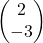or 2i – 3j, which both mean 2 units to the right and 3 down.

You’ll have seen column vector notation at GCSE but i, j notation is new at A-level; i is the unit vector in the x-direction and j is the unit vector in the y-direction. Note that i and j are shown in bold type, or underlined if handwritten, because they are vectors. The two forms are interchangeable, and if a question gives information in i, j form but you prefer to work with column vectors then it’s absolutely fine to use column vector format in your answer. You don’t even have to change it back at the end!

## Finding a resultant force

Finding the resultant of a set of forces is simply a matter of adding together the vectors representing each of the forces. This is covered in the Foundation GCSE post linked above, but we’ll cover it again here.

As with any other vectors, we can find the resultant of a set of forces by placing them end to end. However, if you have the forces in component form then it’s usually easier to consider the perpendicular components acting in each direction separately. This is called resolving the forces.

### Example:

Find the resultant of the forces (3i + 6j) N, (4i – 11j) N and (–5i + 2j) N.

Resolving horizontally (i.e. adding up the i-components), we have 3 + 4 – 5 = 2

Resolving vertically, we have 6 – 11 + 2 = -3

So the resultant is (2i – 3j) N.

If you were to place the vectors end-to-end on a grid then it would look like this (the resultant vector is shown in red):

### Resultant force practice questions

Find the resultants of the following sets of forces:

a) (3i + j) N, (5i – 6j) N and (–8i – 2j) N

b)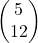N,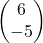N and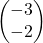N

## Forces in equilibrium

In some cases you will be told that a system is in equilibrium, but one of the forces will be unknown and it will be up to you to work it out. The question you need to ask is, what do the components of this force need to be, in order for all the forces to add up to zero?

(Incidentally, a vector that creates equilibrium in a system is sometimes described as the equilibrant.)

### Example

A particle is held in equilibrium by three forces: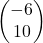N,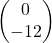N and F N. Find F.

This sketch shows the two known forces, roughly in proportion. Before scrolling down further, try to visualise where the third force would need to be to keep the particle in equilibrium. If you were to place the two known forces end to end, what would the third force need to look like to form a closed triangle?

(It’s almost always a good idea to start off with a sketch, even if it isn’t actually required as part of the answer.)

Your prediction should look something like this:

Let’s describe F using 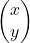.

Since the forces are in equilibrium, they must add up to zero: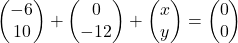Looking at the horizontal components, x = 0 + 6 = 6
and for the vertical components, y = 12 – 10 = 2

So F =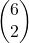.

### Forces in equilibrium practice questions

Find the force F that would hold each system in equilibrium:

a) (9i + 4j) N, (4i – 6j) N and F N

b)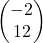N,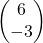N,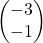N and F N

## Magnitude-direction form of a vector

Sometimes you’ll need to find the magnitude and/or direction of a vector – for example you might be asked for the magnitude of a resultant force, or if you have a velocity in vector form and are asked for the speed then you need to find the magnitude of the velocity vector.

The magnitude (or modulus) is found using Pythagoras, and the direction is usually given as an angle of between -180° and 180° with the positive i-direction (i.e. the direction of the positive x-axis).

Vectorcan be written in magnitude-direction form as (r, θ°) where r is the magnitude and θ is the angle with the positive i-direction.
(In some cases, e.g. where bearings are involved, you’ll need to find a different angle; just make sure that the angle you give is the one you’ve been asked for!)

Always sketch a triangle so that you can see which direction the force is acting in. Include the axes to begin with; once you get more confident you can leave them out if you like.

### Examples

For each of the examples above:

First, use Pythagoras’ theorem to find the magnitude (length of the line).

Then, to find the direction:
a) label the angle θ in the right-angled trangle at the vertex where it touches the origin;
b) label the sides of the triangle and use SOHCAHTOA to find the angle (ignore negative signs);
c) identify the angle between the positive i-direction and the vector. Remember, if the vector is above the x-axis then the angle is positive; if below then it’s negative.

Finally, express the vector in magnitude-direction format.

What is the angle between the forces in (a) and (b)?

## Using Newton’s laws with forces in vector form

Newton’s first law states that a body will remain at rest or move with constant velocity unless acted upon by a resultant force.

So if the system is in equilibrium then the body will remain at rest if it was already stationary, or will keep moving with the same constant velocity if it was already in motion.

Newton’s second law is usually given in the form F = ma, sometimes known as the equation of motion, where F is the resultant force acting on the body, m is its mass and a is its acceleration.

Mass is a scalar quantity but force and acceleration are both vectors, hence the bold type for F and a. When handwritten, they should of course be underlined (if you’re dealing with vectors).

### Example:

A particle of mass 5kg is acted on by a force of (35i – 12j) N. Find:
a) the magnitude and direction of its acceleration;
b) its speed after 4 seconds, if it was initially at rest.

#### Solution:

##### Part (a):

F = ma gives us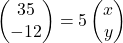where x and y are the components of the acceleration.

Resolving horizontally, 35 = 5x
=> x = 35 ÷ 5 = 7

Resolving vertically, -12 = 5y
=> y = -12 ÷ 5 = -2.4

so a =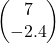ms‾²

Sketch the triangle:

Magnitude of a (write as |a| or simply a) =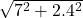= 7.4 ms‾²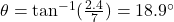and vector is below x-axis
So angle with positive i-direction = -18.9°

##### Part (b):

Acceleration is the gradient of the velocity-time graph, or of the speed-time graph if no vectors are involved.

In this case we’re only looking for speed so we can use just the magnitude of the acceleration.
Acceleration is constant so it’s a straight-line graph,
so acceleration =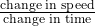.

Since the initial speed was 0 (the body started from rest), this simplifies to
speed = acceleration × time

So speed after 4 seconds = 7.4 × 4 = 29.6 ms‾¹.

(Alternatively you could have worked out the velocity in vector form and just found its magnitude – the speed – at the end.)

### Using F=ma with vectors – practice questions

1) A particle of mass 3 kg is accelerating with vector (4i – 3j) ms‾². Find:
a) the resultant force acting on it in component form;
b) the magnitude and direction of the resultant force.

2) A particle of mass 8kg is acted on by a force of 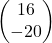N.
a) Find the acceleration of the particle in component form.
b) Find the magnitude of the acceleration.
c) Given that the particle was initially at rest, find its speed after 5 seconds.

3) A particle of mass m kg, initially at rest, is acted on by a force of (4i – 10j) N. Given that its velocity after 3 seconds is (24i – 60j) ms‾¹, find m.

That’s it for the Year 1 content on resultant forces and forces in equilibrium. I hope you’ve found it helpful.

In the second year you’re more likely to be given the magnitude and angle of the force, so you need to work out for yourself what the perpendicular component parts are. This is referred to as resolving a force into its components, and will be covered in a future blog post.

If you’ve found this article helpful then please share it with anyone else who you think would benefit (use the social sharing buttons if you like). If you have any suggestions for improvement or other topics that you’d like to see covered, then please comment below or drop me a line using my contact form.

On my sister site at at mathscourses.co.uk you can find – among other things – a great-value suite of courses covering the entire GCSE (and Edexcel IGCSE) Foundation content, and the “Flying Start to A-level Maths” course for those who want to get top grades at GCSE and hit the ground running at A-level – please take a look!

#### Finding a resultant force

a)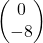N; b)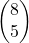N

Back to this section

#### Forces in equilibrium

a)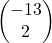N; b)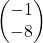N

Back to this section

#### Magnitude-direction format

a)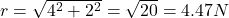;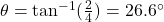Angle with positive i-direction = 26.6°
(4.47 N, 26.6°)

b)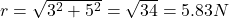;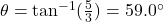Angle with positive i-direction = -59.0°
(5.83 N, -59.0°)

c)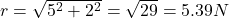;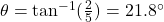Angle with positive i-direction = 180 – 21.8 = 158.2°
(5.39 N, 158.2°)

Angle between (a) and (b) = 26.6 + 59.0 = 85.6°

Back to this section

#### Using F=ma

1a)  N; b) (15 N, –36.7°)
2a) 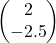ms‾²; b) 3.20 ms‾²; c) 16.0 ms‾¹
3) 0.5 kg

Back to this section